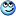# Question on finding maximum magnitude of acceleration

• RoboNerd
In summary, the object's maximum acceleration occurs when its x and y coordinates are both equal to 45 m/s2.

## Homework Statement

An object is moving in the xy-plane according to the equations x(t) = 3sin(3t) and y(t) = 4cos(3t). What is the maximum magnitude of the particle's acceleration?

1. 1) 5 m/s2

2. 2) 15 m/s2

3. 3) 30 m/s2

4. 4) 36 m/s2 [the accepted answer]

5. 5) 45 m/s2

## Homework Equations

x(t) = Asin(wt + phase constant)

## The Attempt at a Solution

So, I know that for each dimension if I take the second derivative of the two position equations I get acceleration, and the maximum acceleration for each of those two is simply A*omega^2.

So that's what I did.

For acceleration in the x-dimension, I get: 3*3^2.
For acceleration in the y-dimension, I get 4*3^2.

Taking the squares of the two acceleration components and then summing them and taking the square root of the sum gives 45, which is what I got. Why do the authors then believe 36 m/sec^2 is the right answer?

Thanks in advance for the input!

Does ax, max occur at the same instant that ay, max occurs?

•cnh1995
no, of course not. how would I fix for that, then?

But how would I be able to tell if the aymax and axmax occur simultaneously?

Can you find an expression for the magnitude of the (total) acceleration as a function of time?

Yes, take the squares of the two equations, sum and square root.

I am too lazy to write it out here, unless it is really needed. ;-)

RoboNerd said:
But how would I be able to tell if the aymax and axmax occur simultaneously?
Find expressions for ax and ay as functions of time. By inspection you will see that their max values do not occur at the same time.

RoboNerd said:
For acceleration in the x-dimension, I get: 3*3^2.
For acceleration in the y-dimension, I get 4*3^2.

i think there are cosine /sine terms also in the accelerations.
check.
or you can find r the radius vector and find out the acceleration and find out the maximum.

Aha, OK. thanks.
TSny said:
Find expressions for ax and ay as functions of time. By inspection you will see that their max values do not occur at the same time.

drvrm said:
i think there are cosine /sine terms also in the accelerations.
check.
or you can find r the radius vector and find out the acceleration and find out the maximum.

I was just doing manipulations of maximum and minimum values [just the amplitudes of the two equations... if you know what I mean]

RoboNerd said:
Yes, take the squares of the two equations, sum and square root.

I am too lazy to write it out here, unless it is really needed. ;-)
That's pretty lazy.I wouldn't have suggested it unless I thought it was worth the trouble.•RoboNerd
sqrt( 3sin(3t)^2 + 4cos(3t)^2 )

Here you go, sir!

No, that's not the sum of the squares of the acceleration components.
##a = \sqrt{a_x^2+a_y^2}##